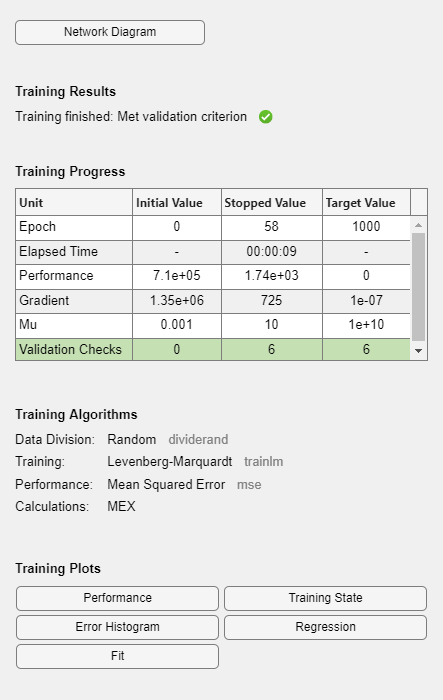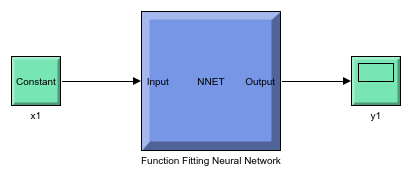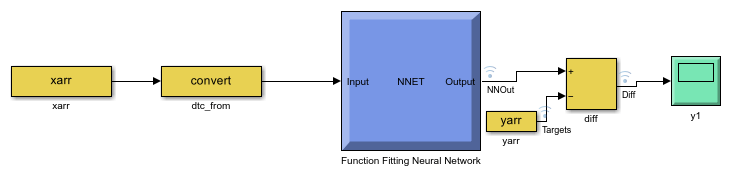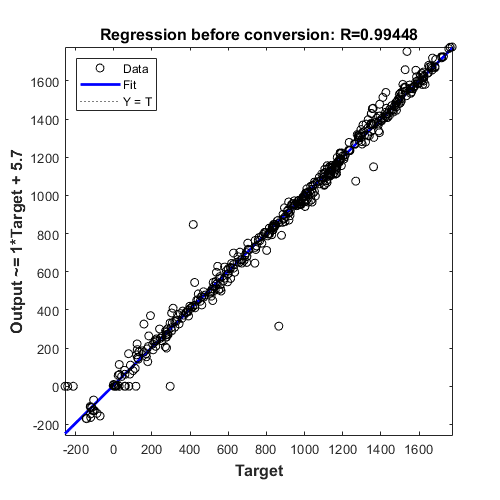# Convert Neural Network Algorithms to Fixed Point and Generate C Code

This example shows how to convert a neural network regression model in Simulink to fixed point using the Fixed-Point Tool and Lookup Table Optimizer and generate C code using Simulink Coder.

### Overview

Fixed-Point Designer provides workflows via the Fixed Point Tool that can convert a design from floating-point data types to fixed-point data types. The Lookup Table Optimizer generates memory-efficient lookup table replacements for unbounded functions such as `exp` and `log2`. Using these tools, this example showcases how to convert a trained floating-point neural network regression model to use embedded-efficient fixed-point data types.

### Data and Neural Network Training

The `engine_dataset` contains data representing the relationship between the fuel rate, speed of the engine, and its torque and gas emissions.

```load engine_dataset; ```

Use the function fitting tool `nftool` from Deep Learning Toolbox™ to train a neural network to estimate torque and gas emissions of an engines given the fuel rate and speed. Use the following commands to train the neural network.

```x = engineInputs; t = engineTargets; net = fitnet(10); net = train(net,x,t); view(net) ```Close the view of the network.

```nnet.guis.closeAllViews(); ```

### Model Preparation for Fixed-Point Conversion

Once the network is trained, use the `gensim` function from the Deep Learning Toolbox™ to generate a simulink model.

```sys_name = gensim(net, 'Name', 'mTrainedNN'); ```The model generated by the `gensim` function contains the neural network with trained weights and biases. To prepare this generated model for fixed-point conversion, follow the preparation steps in the best practices guidelines. https://www.mathworks.com/help/fixedpoint/ug/best-practices-for-using-the-fixed-point-tool-to-propose-data-types-for-your-simulink-model.html

After applying these principles, the trained neural network is further modified to enable signal logging at the output of the network, add input stimuli and verification blocks.

Open and inspect the model.

```model = 'ex_fxpdemo_neuralnet_regression'; system_under_design = [model '/Function Fitting Neural Network']; baseline_output = [model '/yarr']; open_system(model); ```To open the Fixed-Point Tool, right click on the Function Fitting Neural Network subsystem and select `Fixed-Point Tool`. Alternatively, use the command-line interface of the Fixed-Point Tool. Fixed Point Tool and the command-line interface provide workflow steps for model preparation for fixed point conversion, range and overflow instrumentation of objects via simulation and range analysis, homogeneous wordlength exploration for fixed point data typing and additional overflow diagnostics.

```converter = DataTypeWorkflow.Converter(system_under_design); ```

### Run Simulation to Collect Ranges

Simulate the model with instrumentation to collect ranges. This is achieved by clicking the Collect Ranges button in the tool or the following commands.

```converter.applySettingsFromShortcut('Range collection using double override'); % Save simulation run name generated as collect_ranges. This run name is used in % later steps to propose fixed point data types. collect_ranges = converter.CurrentRunName; sim_out = converter.simulateSystem(); ```

Plot the regression accuracy before the conversion.

```plotRegression(sim_out, baseline_output, system_under_design, 'Regression before conversion'); ```### Propose Fixed-Point Data Types

Range information obtained from simulation can be used by the Fixed-Point Tool to propose fixed-point data types for blocks in the system under design. In this example, to ensure that the tools propose signed data types for all blocks in the subsystem, disable the `ProposeSignedness` option in the `ProposalSettings` object.

```ps = DataTypeWorkflow.ProposalSettings; ps.ProposeSignedness = false; converter.proposeDataTypes(collect_ranges, ps); ```

### Apply Proposed Data Types

By default, the Fixed-Point Tool applies all of the proposed data types. Use the `applyDataTypes` method to apply the data types. If you want to only apply a subset of the proposals, in the Fixed-Point Tool use the Accept check box to specify the proposals that you want to apply.

```converter.applyDataTypes(collect_ranges); ```

### Verify Data Types

Proposed types should handle all possible inputs correctly. Set the model to simulate using the newly applied types, simulate the model, and observe that the neural network regression accuracy is retained post fixed-point conversion.

```converter.applySettingsFromShortcut('Range collection with specified data types'); sim_out = converter.simulateSystem(); ```

Plot the regression accuracy of the fixed-point model.

```plotRegression(sim_out, baseline_output, system_under_design, 'Regression after conversion'); ```### Replace Activation Function With an Optimized Lookup Table

The Tanh Activation function in Layer 1 can be replaced with either a lookup table or a CORDIC implementation for more efficient fixed-point code generation. In this example, we will be using the Lookup Table Optimizer to get a lookup table as a replacement for `tanh`. We will be using `EvenPow2Spacing` for faster execution speed. For more information, see https://www.mathworks.com/help/fixedpoint/ref/functionapproximation.options-class.html.

```block_path = [system_under_design '/Layer 1/tansig']; p = FunctionApproximation.Problem(block_path); p.Options.WordLengths = 16; p.Options.BreakpointSpecification = 'EvenPow2Spacing'; solution = p.solve; solution.replaceWithApproximate; ```
```| ID | Memory (bits) | Feasible | Table Size | Breakpoints WLs | TableData WL | BreakpointSpecification | Error(Max,Current) | | 0 | 64 | 0 | 2 | 16 | 16 | EvenPow2Spacing | 7.812500e-03, 1.000000e+00 | | 1 | 16416 | 1 | 1024 | 16 | 16 | EvenPow2Spacing | 7.812500e-03, 1.525879e-03 | | 2 | 8224 | 1 | 512 | 16 | 16 | EvenPow2Spacing | 7.812500e-03, 5.981445e-03 | Best Solution | ID | Memory (bits) | Feasible | Table Size | Breakpoints WLs | TableData WL | BreakpointSpecification | Error(Max,Current) | | 2 | 8224 | 1 | 512 | 16 | 16 | EvenPow2Spacing | 7.812500e-03, 5.981445e-03 | ```

Verify model accuracy after function approximation replacement.

```converter.applySettingsFromShortcut(converter.ShortcutsForSelectedSystem{2}); sim_out = converter.simulateSystem; ```

Plot regression accuracy.

```plotRegression(sim_out, baseline_output, system_under_design, 'Regression after function replacement'); ```### Generate C Code

To generate C code using Simulink Coder™, right-click on the Function Fitting Neural Network subsystem, select C/C++ Code > Build Subsystem, then click the Build button when prompted for tunable parameters. You can also generate code by using the following command. `slbuild('fxpdemo_neuralnet_regression_toconvert/Function Fitting Neural Network')`

## SupportGet trial now